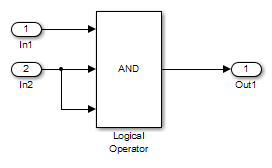## Logic Operations Short-Circuiting

Simulink® Design Verifier™ considers logical operations and logical expressions as short-circuiting when analyzing for dead logic and when generating tests.

Logical Operators and Logical Expressions for `Condition` and `MCDC` objectives can be considered short-circuiting or not when you analyze for dead logic or generate tests. The table summarizes different considerations:

Short-Circuit consideration for `Condition` or `MCDC` Objectives

Modeling elementShort-Circuit consideration for `Condition` or `MCDC` Objectives

MATLAB®, Stateflow® (C/MATLAB) and other Simulink Blocks (Fcn, If)

Always short-circuited

Short-circuited only when `CovLogicBlockShortCircuit` is ON

For `Condition` objective, consider the following simple logical operator example model. When `CovLogicBlockShortCircuit` parameter is ON, a previous input alone determines the block output, the analysis ignores any remaining block inputs. If the first input to a Logical Operator block whose Operator parameter specifies `AND` is false, the analysis ignores the values of the other inputs.

When `CovLogicBlockShortCircuit` parameter is OFF, all the inputs are considered.The tables summarizes the difference in objectives for short-circuit and non short-circuit case for `Condition` objective by using a similar logical expression for MATLAB function block:

Short-Circuit considerations for `Condition` objective

Condition ‘F’ Port 3`CovLogicBlockShortCircuit`: ON`CovLogicBlockShortCircuit`: OFF

Logical operator

(in1) && (in2) && (~in2) (dead logic)

~ in2 (active logic)

MATLAB Function with logical expression (in1 && in2 && in2)

(in1) && (in2) && (~in2) (dead logic)

(in1) && (in2) && (~in2) (dead logic)

For `MCDC` objective, along with the short-circuit mode, the `CovMCDCMode` or the coverage MCDC mode is set as a parameter.

The tables summarizes the difference in objectives for short-circuit and non short-circuit case for `MCDC` objective.

Short-Circuit considerations for `MCDC` objective

`CovLogicBlockShortCircuit``CovMCDCMode`Standalone blockCascaded Network

ON

Short circuited `MCDC`

`MCDC` for network (Short-circuited)

OFF

Non short-circuited `MCDC`

`MCDC` for network (Non short-circuited)

ON

Unique cause

Short-circuited `MCDC`

`MCDC` (Short-circuited) coverage result can differ from Simulink Design Verifier.

OFF

Unique cause

Non short-circuited `MCDC`

NA

Note

If `covMCDCMode` is Unique cause, then `MCDC` definition differs between coverage and `MCDC`.